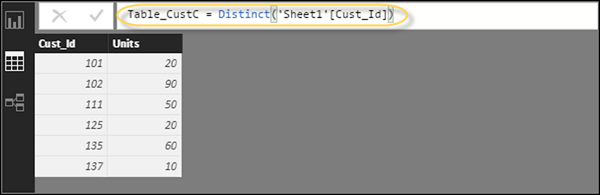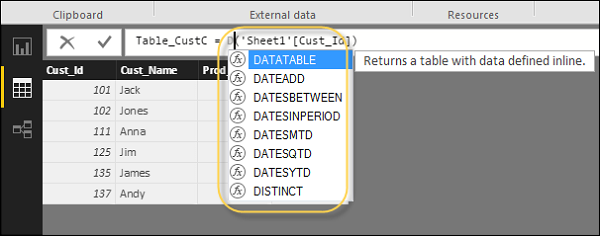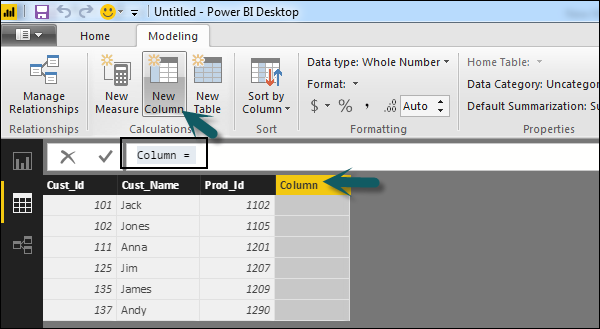# DAX Basics in Power BI

In this chapter, you will learn how to use various DAX functions in Power BI.

## DAX Introduction

DAX (Data Analysis Expressions) is a formula expression language and can be used in different BI and visualization tools. DAX is also known as function language, where the full code is kept inside a function. DAX programming formula contains two data types: Numeric and Other. Numeric includes - integers, currency and decimals, while Other includes: string and binary object.

Following is an example of DAX formula, which uses a function to calculate a column in a table.DAX function can also include other functions, conditional statements, and value references.

## DAX Functions

In Power BI, you can use different function types to analyze data, and create new columns and measures. It includes functions from different categories such as −

• Aggregate
• Text
• Date
• Logical
• Counting
• Information

Power BI provides an easy way to see the list of all functions. When you start typing your function in the formula bar, you can see the list of all functions starting with that alphabet.### Aggregate Functions

DAX has a number of aggregate functions.

• MIN
• MAX
• Average
• SUM
• SUMX

### Counting Functions

Other counting functions in DAX include −

• DISTINCTCOUNT
• COUNT
• COUNTA
• COUNTROWS
• COUNTBLANK

### Logical Functions

Following are the collection of Logical functions −

• AND
• OR
• NOT
• IF
• IFERROR

• REPLACE
• SEARCH
• UPPER
• FIXED
• CONCATENATE

• DATE
• HOUR
• WEEKDAY
• NOW
• EOMONTH

• ISBLANK
• ISNUMBER
• ISTEXT
• ISNONTEXT
• ISERROR

## DAX Calculation Types

In Power BI, you can create two primary calculations using DAX −

• Calculated columns
• Calculated measures

When you navigate to the Modeling tab, you can see a New Column option at the top of the screen. This also opens the formula bar where you can enter DAX formula to perform the calculation. DAX - Data Analysis Expression is a powerful language used in Excel to perform calculations. You can also rename the column by changing the Column text in the formula bar.In the following example, we have created a new column: Product Code (Product_C), which is derived from the last 3 characters of Prod_Id column. Following is the formula −

```Product_C = RIGHT( Sheet1[Prod_Id],3)
```

To create a calculated measure, navigate to New Measure tab under Modeling. This will add a new object under the Fields tab with the name Measure.You can write DAX formula to calculate the value of the new measure, as we did for the new calculated column.